# Mycobacterium Leprae Metabolic Network

Metabolic cellular network data

## Examples

### Basic Examples

Retrieve the graph:

 In:=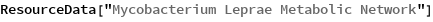Out=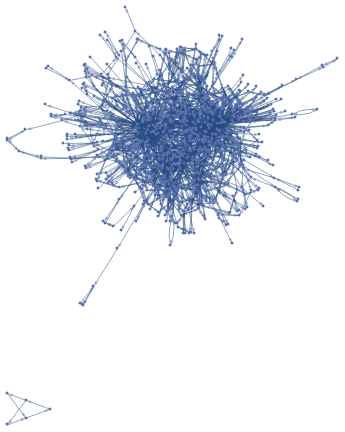Summary properties:

 In:=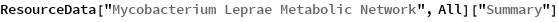Out=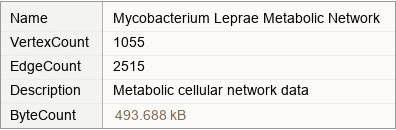### Basic Applications

Create a histogram of the centrality:

 In:=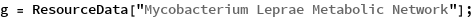In:=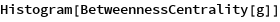Out=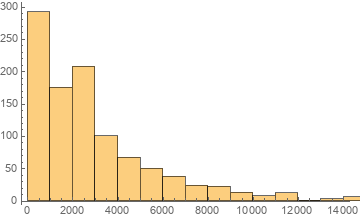Show a table of properties:

 In:=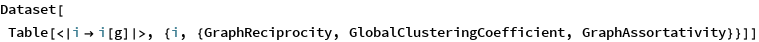Out=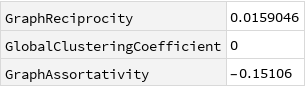Wolfram Research, "Mycobacterium Leprae Metabolic Network" from the Wolfram Data Repository (2019)